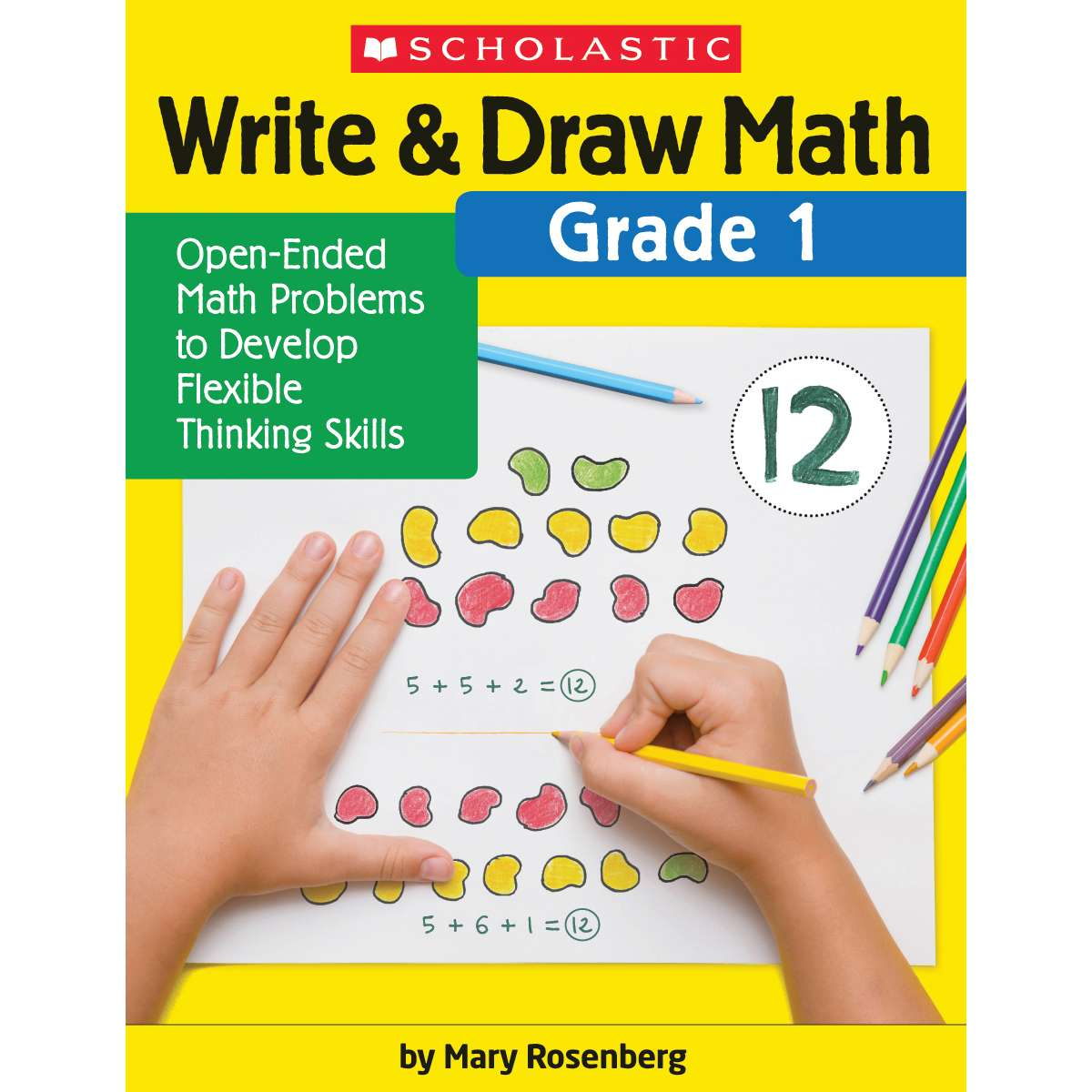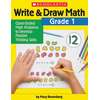•# Write & Draw Math, Grade 1

Product Number: SC-831437
\$11.99
How many ways can you answer a math question? In this book filled with open-ended problems, there is no one correct answer. C...
Quantity

How many ways can you answer a math question? In this book filled with open-ended problems, there is no one correct answer. Children learn to look at the same math problem in different ways and solve it using manipulatives, drawing, or writing. Because there is no single correct answer, each reproducible activity page can be reused multiple times as children’s math skills grow throughout the year. A fun and engaging way to promote mathematical thinking!
SC-831437